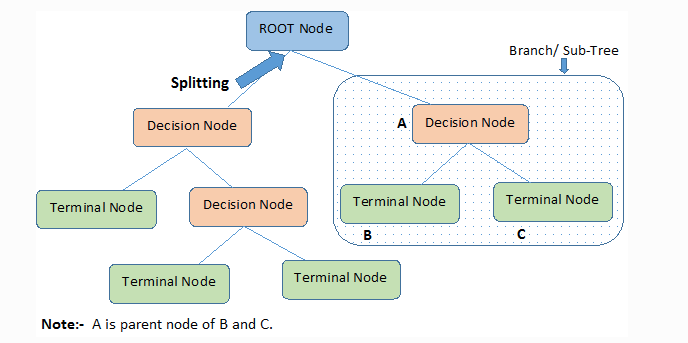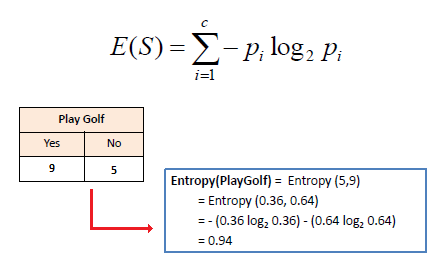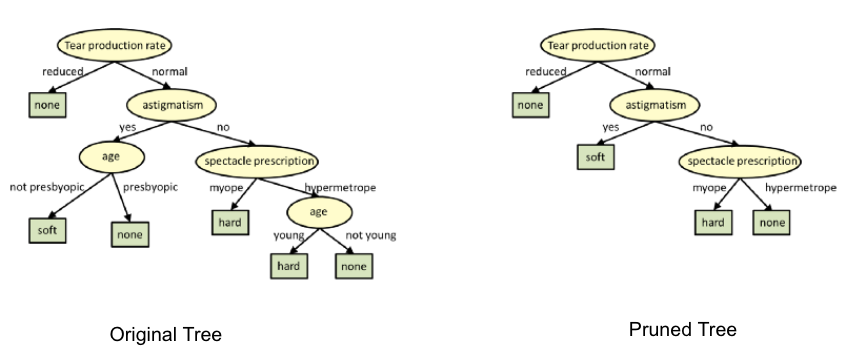# Decision Tree

Decision Tree algorithm is a part of supervised learning algorithms.

It is used for both regression and classification.

1. Root Node: It represents the entire population or sample and this further gets divided into two or more homogeneous sets.
2. Splitting: It is a process of dividing a node into two or more sub-nodes. Decision Node: When a sub-node splits into further sub-nodes, then it is called the decision node.
3. Leaf / Terminal Node: Nodes do not split is called Leaf or Terminal node.
4. Pruning: When we remove sub-nodes of a decision node, this process is called pruning. You can say the opposite process of splitting.
5. Branch / Sub-Tree: A subsection of the entire tree is called branch or sub-tree.
6. Parent and Child Node: A node, which is divided into sub-nodes is called a parent node of sub-nodes whereas sub-nodes are the child of a parent node.## Workflow of a Decision Tree​

Decision tree works based on node split strategy.

The different measures of splitting the nodes of a decision tree are -

• Entropy - It is the measure of randomness in the features.• Information Gain - measures how well an attribute is separated.

##### note

We want highest information gain and lowest entropy

Information gain = Entropy before split - Entropy after split

• Gini Index - It is a cost function used to evaluate performance.

Gini = $1 - \sum{(p_i)^2}$

where, pi = probabilities of each class.

##### note

Higher value of Gini Index -> Higher iequality, heterogeneity.

• Pruning - Trimming of the branches of a tree or removing decision nodes from the root note such that the overall accuracy is not disturbed.